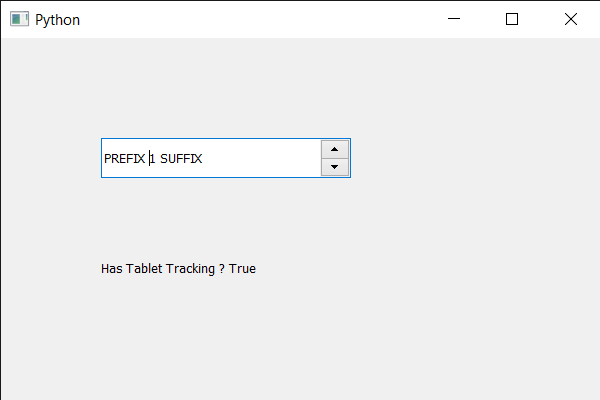Open in App
Not now

# PyQt5 QSpinBox – Setting tablet tracking

• Last Updated : 28 May, 2020

In this article we will see how we can set tablet tracking to the spin box. By default tablet tracking is in disabled state although we can change this any time. If tablet tracking is switched off, tablet move events only occur if the stylus is in contact with the tablet, or at least one stylus button is pressed, while the stylus is being moved. If tablet tracking is switched on, tablet move events occur even while the stylus is hovering in proximity of the tablet, with no buttons pressed.

In order to do this we use `setTabletTracking` method with the spin box object.

Syntax : spin_box.setTabletTracking(True)

Argument : It takes bool as argument

Return : It returns None

Below is the implementation

 `# importing libraries``from` `PyQt5.QtWidgets ``import` `*` `from` `PyQt5 ``import` `QtCore, QtGui``from` `PyQt5.QtGui ``import` `*` `from` `PyQt5.QtCore ``import` `*` `import` `sys`` ` ` ` `class` `Window(QMainWindow):`` ` `    ``def` `__init__(``self``):``        ``super``().__init__()`` ` `        ``# setting title``        ``self``.setWindowTitle(``"Python "``)`` ` `        ``# setting geometry``        ``self``.setGeometry(``100``, ``100``, ``600``, ``400``)`` ` `        ``# calling method``        ``self``.UiComponents()`` ` `        ``# showing all the widgets``        ``self``.show()`` ` `        ``# method for widgets``    ``def` `UiComponents(``self``):``        ``# creating spin box``        ``self``.spin ``=` `QSpinBox(``self``)`` ` `        ``# setting tablet tracking to the spin box``        ``self``.spin.setTabletTracking(``True``)`` ` `        ``# setting geometry to spin box``        ``self``.spin.setGeometry(``100``, ``100``, ``250``, ``40``)`` ` `        ``# setting range to the spin box``        ``self``.spin.setRange(``1``, ``999999``)`` ` `        ``# setting prefix to spin``        ``self``.spin.setPrefix(``"PREFIX "``)`` ` `        ``# setting suffix to spin``        ``self``.spin.setSuffix(``" SUFFIX"``)`` ` `        ``# creating a label``        ``label ``=` `QLabel(``self``)`` ` `        ``# making the label multi line``        ``label.setWordWrap(``True``)`` ` `        ``# setting geometry to the label``        ``label.setGeometry(``100``, ``200``, ``200``, ``60``)`` ` `        ``# checking if spin box has tablet tracking``        ``check ``=` `self``.spin.hasTabletTracking()`` ` `        ``# setting text to the label``        ``label.setText(``"Has Tablet Tracking ? "` `+` `str``(check))`` ` ` ` `# create pyqt5 app``App ``=` `QApplication(sys.argv)`` ` `# create the instance of our Window``window ``=` `Window()`` ` `# start the app``sys.exit(App.``exec``())`

Output :My Personal Notes arrow_drop_up